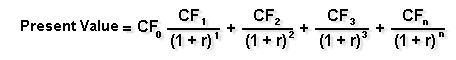Net Present Value of Major Purchases

The net present value method (NPV) of evaluating a major project allows you to consider the time value of money. Essentially, it helps you find the present value in "today's dollars" of the future net cash flow of a project. Then, you can compare that amount with the amount of money needed to implement the project.

If the NPV is greater than the cost, the project will be profitable for you (assuming, of course, that your estimated cash flow is reasonably close to reality). If you have more than one project on the table, you can compute the NPV of both, and choose the one with the greatest difference between NPV and cost.

As an example of how NPV works, let's say you're looking at a project costing \$7,500 that is expected to return \$2,000 per year for five years, or \$10,000 in total. At first glance, the project looks profitable. Under the payback method, it looks as if the project will pay for itself in 3.75 years. However, using NPV analysis you can determine that if the discount rate on the project was 10 percent, the net present value of the expected returns would be \$7,581.60. In other words, if you had \$7,581.60 today and invested it at 10 percent, after five years you'd wind up with \$10,000, the same return as your project. Thus, it looks as if the expected additional return on the project has shrunk to about \$81, which may not be worth all the time and effort you'd have to put in.

NPV analysis is generally used to evaluate the project's cash flows, rather than the income from the project that would be shown on an income statement. Why? Because the income statement factors in depreciation, but depreciation is not an out-of-pocket expense. For instance, if revenue of \$10,000 is reduced to \$7,000 of income because of a \$3,000 depreciation deduction, you still have the use of the full \$10,000. So, the cash flow figure of \$10,000 is the more instructive one to look at. However, if you are very concerned about the appearance of your income statement (for example, if you anticipate putting the business up for sale or seeking major financing in the future, or if you're under stockholder pressure to show more income) you may decide that the income figure is more appropriate to use.

How do you compute NPV? The easiest way is to use a good financial calculator. If you don't have one, or don't want to take the time to learn how to use one, you can use the present value table contained among the Business Tools.Business Tools contains a simple "present value of \$1" table that you can use to figure the NPV of your project.

If you are mathematically inclined and have a calculator with exponential functions, you can also use the following formula:(When using this formula, CFx = cash flow in period x, n = the number of periods, and r = the discount rate.)

Note that whenever you do time value of money calculations to find a present or future value (such as NPV), you'll need to specify an interest rate, known as the discount rate. Choosing the appropriate discount rate is a very important part of the process.